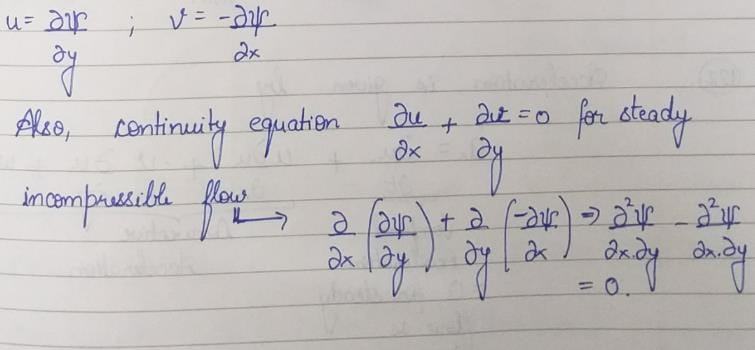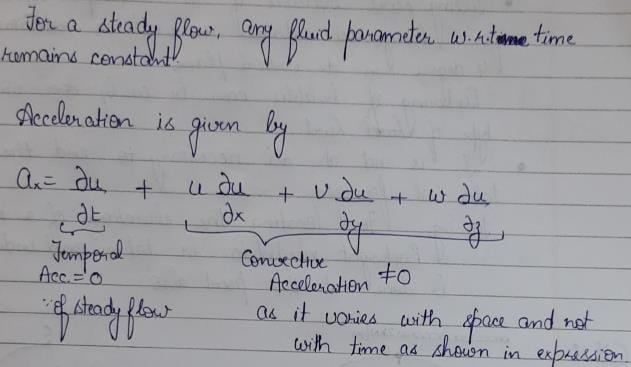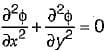Test: Fluid Flow Kinematics - 2

# Test: Fluid Flow Kinematics - 2

Test Description

## 10 Questions MCQ Test GATE Civil Engineering (CE) 2023 Mock Test Series | Test: Fluid Flow Kinematics - 2

Test: Fluid Flow Kinematics - 2 for Civil Engineering (CE) 2023 is part of GATE Civil Engineering (CE) 2023 Mock Test Series preparation. The Test: Fluid Flow Kinematics - 2 questions and answers have been prepared according to the Civil Engineering (CE) exam syllabus.The Test: Fluid Flow Kinematics - 2 MCQs are made for Civil Engineering (CE) 2023 Exam. Find important definitions, questions, notes, meanings, examples, exercises, MCQs and online tests for Test: Fluid Flow Kinematics - 2 below.
Solutions of Test: Fluid Flow Kinematics - 2 questions in English are available as part of our GATE Civil Engineering (CE) 2023 Mock Test Series for Civil Engineering (CE) & Test: Fluid Flow Kinematics - 2 solutions in Hindi for GATE Civil Engineering (CE) 2023 Mock Test Series course. Download more important topics, notes, lectures and mock test series for Civil Engineering (CE) Exam by signing up for free. Attempt Test: Fluid Flow Kinematics - 2 | 10 questions in 30 minutes | Mock test for Civil Engineering (CE) preparation | Free important questions MCQ to study GATE Civil Engineering (CE) 2023 Mock Test Series for Civil Engineering (CE) Exam | Download free PDF with solutions
 1 Crore+ students have signed up on EduRev. Have you?
Test: Fluid Flow Kinematics - 2 - Question 1

### A 2-D flow having velocity V = (x + 2y + 2)i + (4 - y)j will be

Test: Fluid Flow Kinematics - 2 - Question 2

### In a flow net

Test: Fluid Flow Kinematics - 2 - Question 3

### A stream function is

Detailed Solution for Test: Fluid Flow Kinematics - 2 - Question 3

A stream function is defined by following characteristic:
The partial derivative of stream function w.r.t y will give velocity in x-direction.
The partial derivative of stream function w.r.t x will give velocity in negative y-direction.
It is valid for steady, incompressible flow since, is satisfies the continuity equationTest: Fluid Flow Kinematics - 2 - Question 4

The continuity equation for steady incompressible flow is expressed in vector notation as

Test: Fluid Flow Kinematics - 2 - Question 5

In a converging steady flow there is

Detailed Solution for Test: Fluid Flow Kinematics - 2 - Question 5Test: Fluid Flow Kinematics - 2 - Question 6

In two dimensional flow, the equation of a streamline is given as

Test: Fluid Flow Kinematics - 2 - Question 7

The concept of stream function which is based on the principle of continuity is applicable to

Detailed Solution for Test: Fluid Flow Kinematics - 2 - Question 7

Velocity potential function is valid for 3-dimensional flow while stream function is valid for 2 dimensional flow.

Test: Fluid Flow Kinematics - 2 - Question 8

Which of the following velocity potentials satisfies continuity equation?

Detailed Solution for Test: Fluid Flow Kinematics - 2 - Question 8

For the velocity potential function to satisfy continuity equation:Where φ is velocity potential, φ = x2 - y2 satisfies this equation

Test: Fluid Flow Kinematics - 2 - Question 9

In a two dimensional incompressible steady flow around an airfoil, the stream lines are 2 cm apart at a great distance from the airfoil, where the velocity is 30 m/sec. The velocity near the airfoil, where the stream lines are 1.5 cm apart, is

Detailed Solution for Test: Fluid Flow Kinematics - 2 - Question 9

V1y1 = V2y2
⇒ 30 x 2 = V2 x 1.5 ⇒ v2 = 40 cm/s

Test: Fluid Flow Kinematics - 2 - Question 10

The velocity potential function for a source varies with distance r as

Detailed Solution for Test: Fluid Flow Kinematics - 2 - Question 10

Velocity at any point r in the flow field of source is given by, Vr = q/2πr.

## GATE Civil Engineering (CE) 2023 Mock Test Series

27 docs|296 tests
 Use Code STAYHOME200 and get INR 200 additional OFF Use Coupon Code
Information about Test: Fluid Flow Kinematics - 2 Page
In this test you can find the Exam questions for Test: Fluid Flow Kinematics - 2 solved & explained in the simplest way possible. Besides giving Questions and answers for Test: Fluid Flow Kinematics - 2, EduRev gives you an ample number of Online tests for practice

## GATE Civil Engineering (CE) 2023 Mock Test Series

27 docs|296 tests